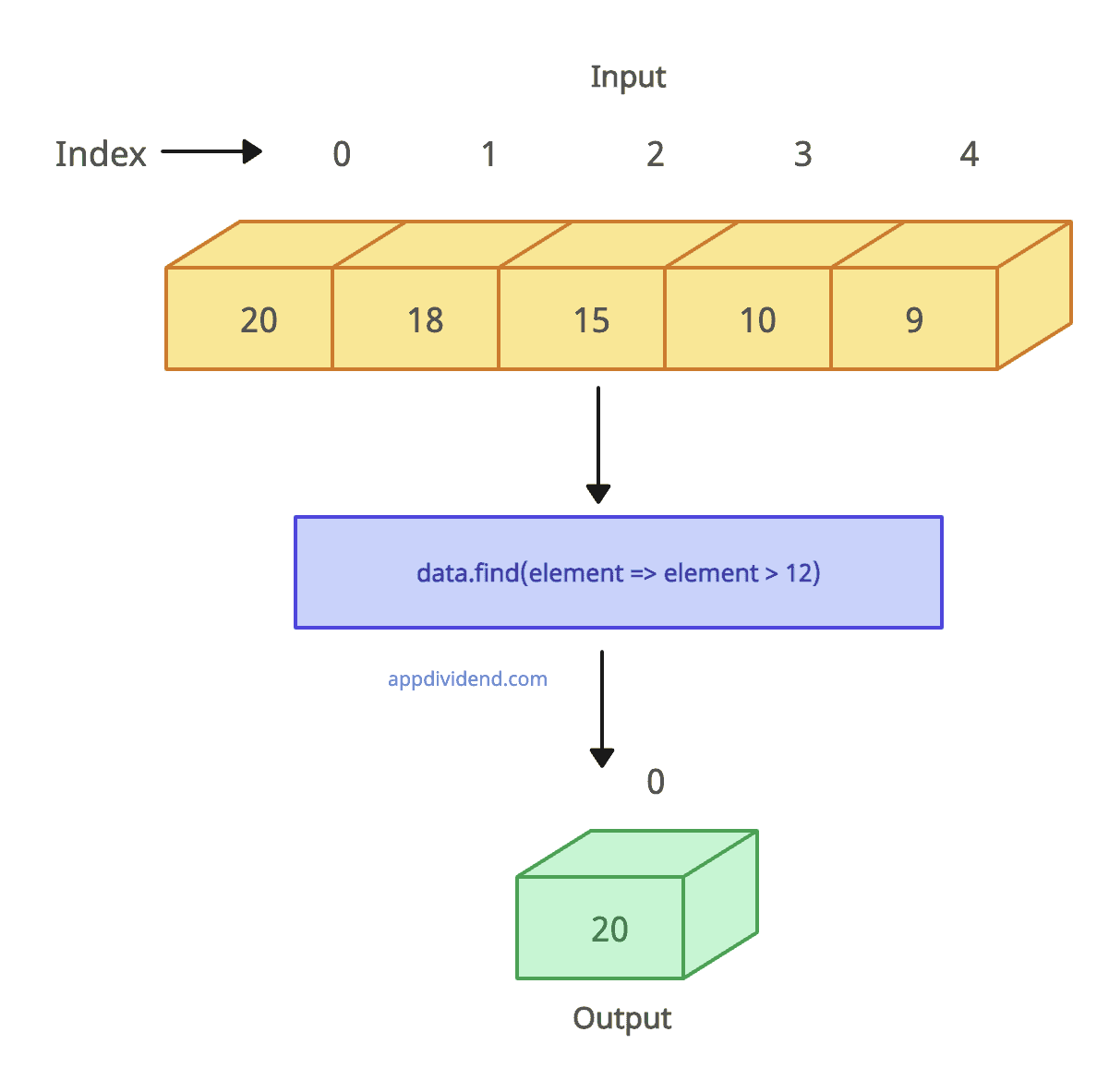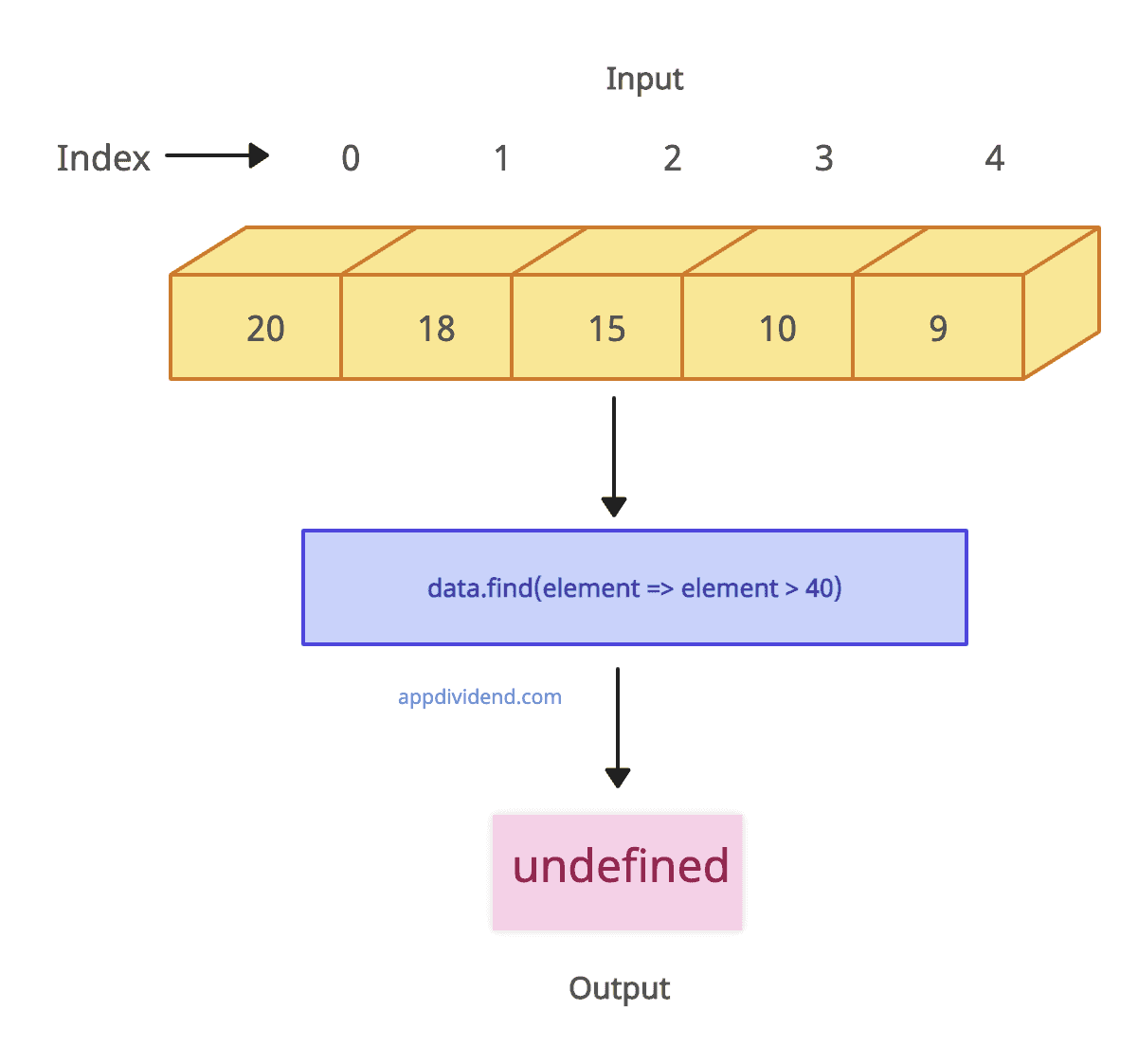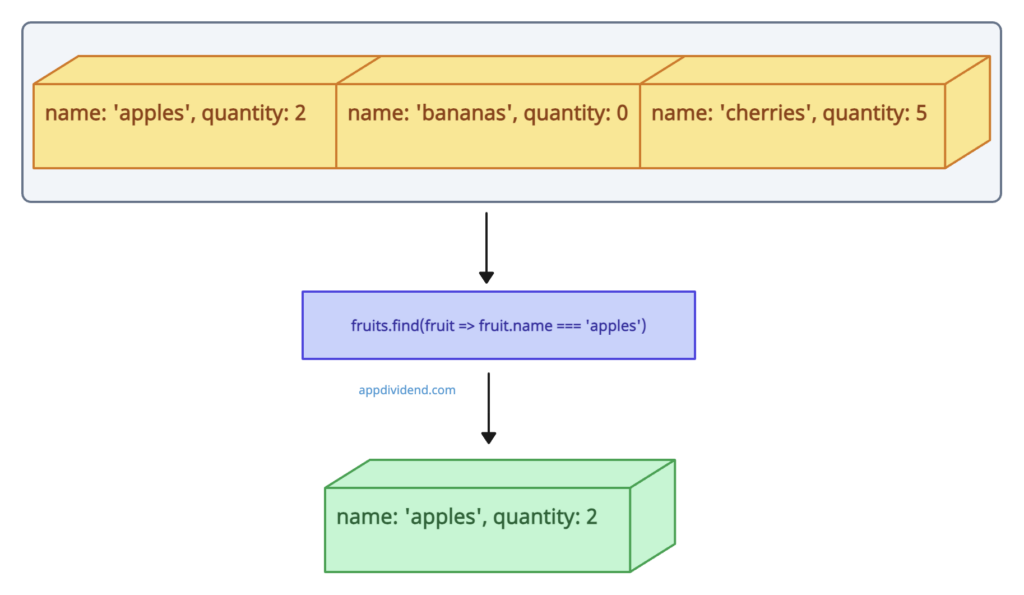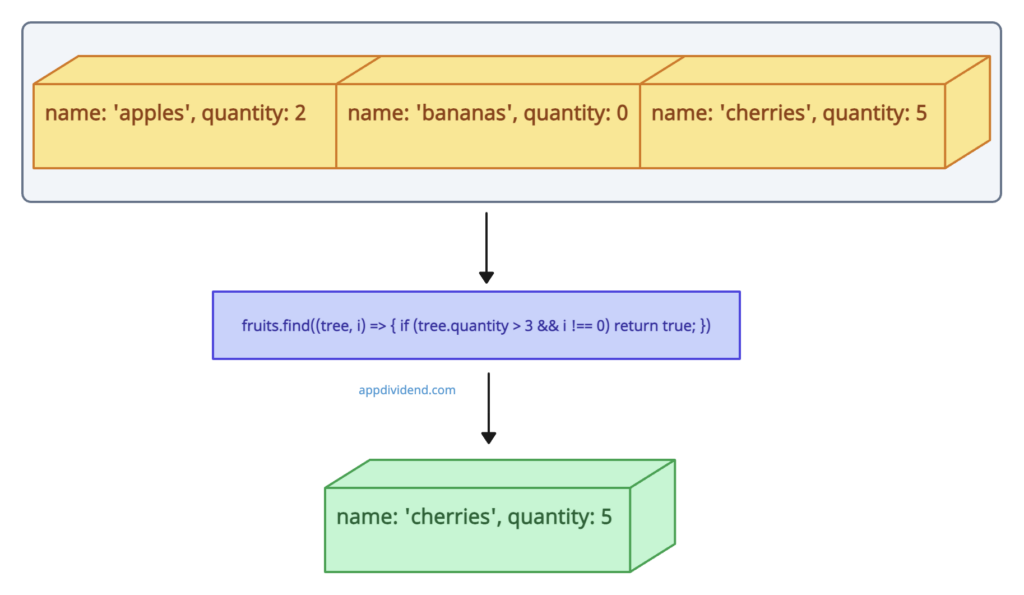# JavaScript Array find() Method

JavaScript Array find() method is used to get a value of the first element in the array that satisfies the provided test function. If no element is satisfied, it returns undefined.

## Syntax

``array.find(function(element, index, arr),thisValue)``

## Parameters

• function(required): This is a function that executes on each element of the array.
• element(required): This is the current item being processed by the function.
• index(Optional): This is the index of the current element processed by the function.
• arr(Optional): The array find was called upon.
• thisValue(Optional): It tells the function to use the array value when executing an argument function.

## Return value

It returns the first element in the array that satisfies the condition, otherwise undefined.

## Example 1: How to Use Array find() Method

``````let data = [20, 18, 15, 10, 9];

let found = data.find(function(element) {
return element < 12;
});

console.log(found);``````

Output

``10``

## Example 2: Passing an Arrow function``````let data = [20, 18, 15, 10, 9];

let found = data.find(element => element > 12);

console.log(found);``````

Output

``20``

## Example 3: No element is found``````let data = [20, 18, 15, 10, 9];

let found = data.find(element => element > 40);

console.log(found);``````

Output

``undefined``

## Example 4: Using an array of objects``````let fruits = [
{name: 'apples', quantity: 2},
{name: 'bananas', quantity: 0},
{name: 'cherries', quantity: 5}
];

let getFruit = fruits.find(fruit => fruit.name === 'apples');

console.log(getFruit);``````

Only one object will be returned if the condition is met successfully.

Output

``{ name: 'apples', quantity: 2 }``

## Example 5: Using the index parameterHere’s how you can use the index parameter:

``````let fruits = [
{name: 'apples', quantity: 2},
{name: 'bananas', quantity: 0},
{name: 'cherries', quantity: 5}
];

let getFruit = fruits.find((tree, i) => { if (tree.quantity > 3 && i !== 0) return true; });

console.log(getFruit);
``````

Output

``{ name: 'cherries', quantity: 5 }``

## Browser compatibility

• Edge 12
• Firefox 25
• Opera 32
• Safari 8

### 1 thought on “JavaScript Array find() Method”

1.it`s a great tutorial thanks